Mathematics

# Graph of Quadratic Function

326 views

Table of Contents

 1 Introduction 2 Graph of $$x^2$$ 3 How to find x intercept of a quadratic function 4 How to find the y intercept of a parabola 5 How to find the vertex of a quadratic function 6 How to graph a quadratic function 7 Summary 8 FAQs

21 December 2020

Read time: 5 minutes

## Introduction

### Solving quadratic equations by graphing

Solving quadratic equations by graphing is preferred since the (real-number) solutions to any equation are the x-intercepts of that equation, we can find out the x-intercepts of the graph and find the solutions to the equation.

However, there are difficulties with solving quadratic equations by graphing.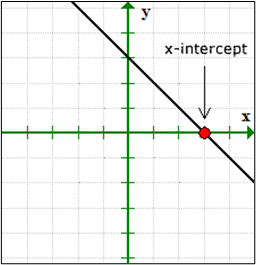It is agreed that solving a quadratic equation is much simpler by solving quadratic equations by graphing. So this blog will help us answer the question “How to graph a quadratic function?”

Quadratic equations of the roots are the x-intercepts of the graph.

Also read:

## Downloadable PDF

Here are some additional points that talk about how to preparing for the exam. To view them click on the Download button.

 📥 Is your child prepared for the future? Download

## Graph of $$x^2$$

The quadratic function graph can be easily derived from the graph of $$x^2.$$

Graph of $$x^2$$ is basically the graph of the parent function of quadratic functions.

A quadratic function is a polynomial and their degree 2 which can be written in the general form,

$f(x)=ax^2+bx+c$

Now, a, b and c expressed real numbers and $$a≠0.$$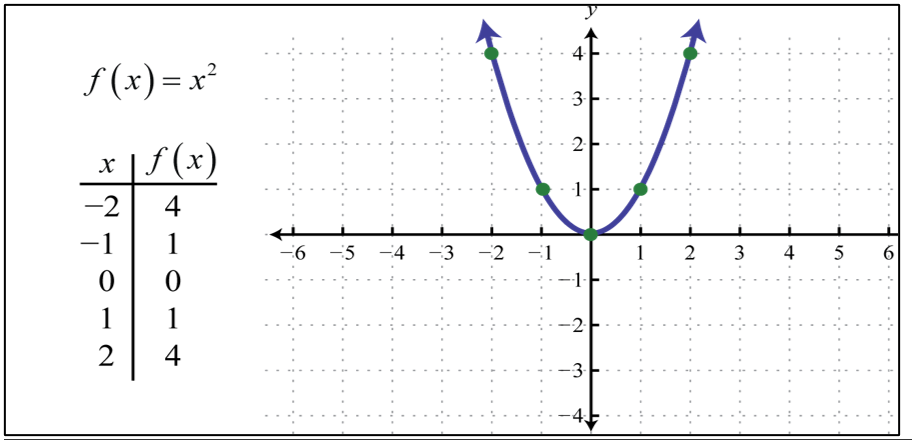This Normally curved shape is called a parabola and it will be shared by all the quadratic function graph.

And this quadratic function graph is indeed a function as it passes the vertical line test. the domain of the function consists of the set of all real numbers (−∞,∞) and the range consists of the set of nonnegative numbers [0,∞).

## How to find x intercept of a quadratic function

We have one more way to find out the quadratic function graph. So, let's come with me and learn How to find x intercept of a quadratic function graph.

The x-intercepts are points where the quadratic function graph passes the x-axis. And the value as a point with a y-value of zero.

So, How to find x intercept of a quadratic function?

• Find the x-intercepts of an equation, and y = 0 then solve for x
• And it is written also as ( x, 0 )

### How to find the zeros of a quadratic function?

The x-intercepts of the function $$f(x)=ax^2+bx+c,\; a ≠ 0$$ are the solutions of the quadratic equation. The solutions of a quadratic equation of the form $$ax^2+bx+c=0$$ are given by the quadratic formula.

$X = \sqrt{−b±b2−\frac{4ac}{2a}}$

### X intercept of a Straight Line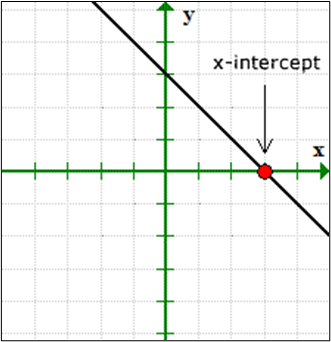### X intercepts of a Quadratic Function or Parabola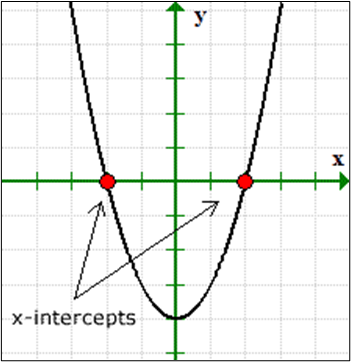## How to find the y intercept of a parabola

Now we can explain the Y-Intercept, lets see how we can explain how to find the y intercept of a parabola.

The y-intercepts are points of a function or an equation that crosses or “touches” the y-axis of the Cartesian Plane. And the point with an x-value of zero.

• find the y-intercepts of an equation, and x = 0 then solve for y.
• it is also written as ( 0, y ).

### Y intercept of a Straight Line

It can be observed in the quadratic function graph.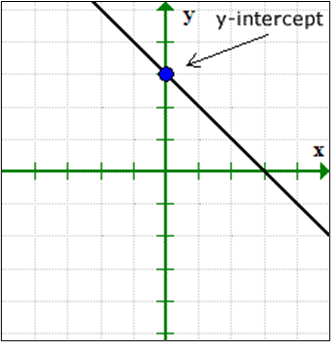### Y intercept of a Quadratic Function graph or Parabola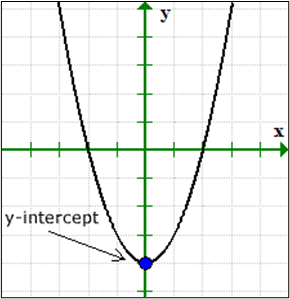Example 1

Describe the x and y-intercepts using point notation.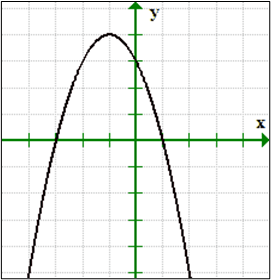Graphs pass the x-axis point at x = 1 and x = 3, therefore, we can write the x-intercepts as points (1,0) and (–3, 0).

Similarly, the graph passes the y-axis at y = 3. And we can write y-intercept as the point ( 0,3 ).

 Example 2

How we can find the x and y-intercepts of the line $$y = –2x + 4.$$

When we find out the x-intercepts, let y = 0 in the equation and then solve for values of x. In the same manner, to find for y-intercepts, let x = 0 in the equation and then solve for y.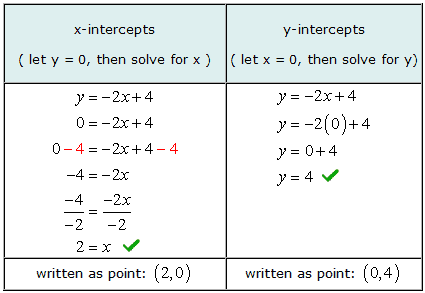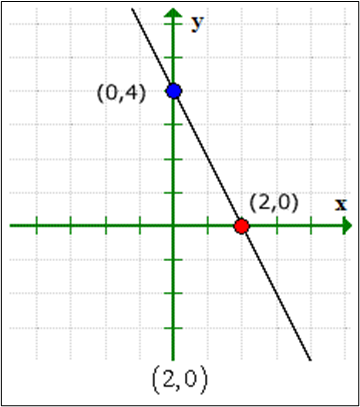## How to find the vertex of a quadratic function?

We have alternate options for how to find the vertex of a quadratic function.

Any quadratic function can be written as:

$X =F (x) = ax^2+bx+c$

In this form, the vertex is (h,k).

To see that this is the case, consider graphing $$f(x)=(x−2)^2+3$$using the transformations.

Using the transformations will help us in answering “how to find the vertex of a quadratic function?”

$$y=x^2$$ Basic Squaring function

$$Y = (x - 2)^2$$ Horizontal shift right 2 units

$$Y = (x - 2)^2+3$$ Vertical shift up 3 units.

Use these translations to sketch the graph,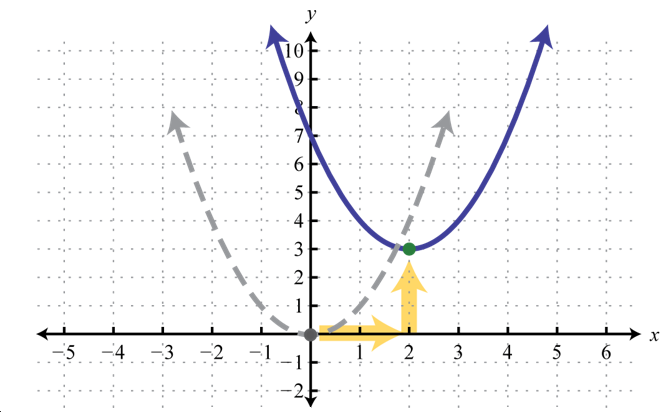Now, we can see the vertex is (2, 3).

\begin{align}f(x) &= a (x-h)^2 + k\\\\f(x) &= a (x-2)^2 + 3 \end{align}

 Example

How to find the vertex of a quadratic function $$f(x)=2 (x+3)−2.$$

Solution:

write the equation before determining h and k.

\begin{align}f(x) &= a (x-h)^2 + k\\\\f(x) &= [2(-x (-3) ]^2 + (-2)\end{align}

Here h = −3 and k = −2.

Answer: The vertex is (−3, −2).

Now let us move onto actually how to graph a quadratic function?

## How to graph a quadratic function?

While quadratic functions can be solved manually using equations as well, solving quadratic functions graphically can make it simpler at times. So let us finally look at how to graph a quadratic function?

A quadratic function is a polynomial of degree 2 which can be written in the general form.

\begin{align}F (x) = ax^2 + bx + c\end{align}

Then, a, b and c represent real numbers where a ≠ 0.

The squaring function $$f ( x ) = x^2$$ is called a quadratic function.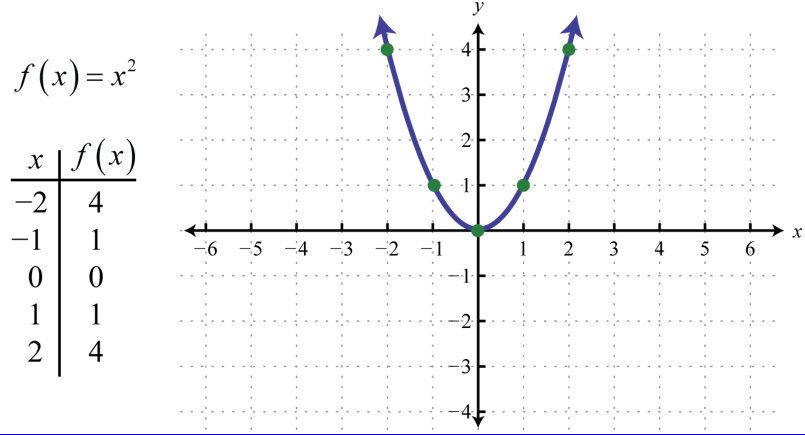This general curved shape is called a parabola.

 Example

How to graph a quadratic function $$f(x) = -x^2 - 2x + 3$$?

Solution:

Step 1  Determine the y-intercept. To do this, set x=0 and find f(0).

\begin{align}f(x) &= - x^2 - 2x + 3\\\\f(x) &= -(0)^2 -2(0) + 3\\\\&= 3\end{align}

The y-intercept is (0,3).

Step 2 Determine the x-intercepts if any. $$f (x) = 0$$ and solve for x.

\begin{align}f(x) &= - x^2 - 2x + 3\\\\0 &= -x^2 -2x +3\\\\0 &= x^2 + 2x +3\\\\0 &= (x+3) (x - 1)\\\\&(x+3 = 0) \quad \text{or} \quad (x- 1 = 0)\\\\&(x= -3 \text{ or } x =1)\end{align}

Step 3 Determine the vertex.

find the x-value of the vertex and then substitute the value of the function to find the corresponding y-value. In this example, a=−1 and b=−2.

\begin{align}x&= \frac{-b}{2a}\\\\x &= \frac{-2}{2(-1)}\\\\x &= \frac{2}{-2} \Rightarrow -1\\\\f(x) &= -x^2 − 2x + 3\\\\f(x) &= -(-1)^2 - 2(-1) +3-1 +2 +3\\\\&= 4\end{align}

The vertex is (−1,4).

Step 4 We have at least five points to plot on either side of the line of symmetry. In this example, one other point will suffice. Choose x=−2 and find the corresponding y-value.

Our fifth point is (−2,3 )

Step 5 Plot the points and draw the graph. The points that we have found are:

Y intercept :- (0,3)

X intercepts :- (-3,0) and (1,0)

Vertex :- (-1, 4)

Extra Point :- (-2,3)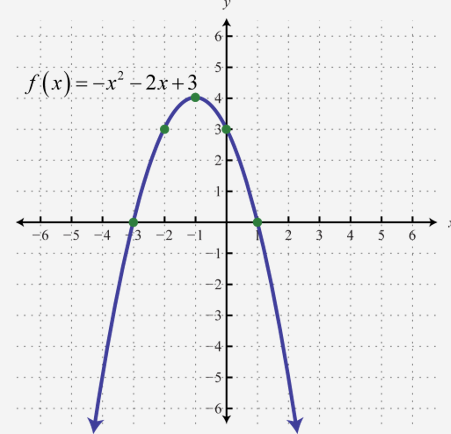The coefficient is to determine if the parabola opens upward or downward. When the coefficient is negative, as in the previous example, then the parabola opens downward. when the coefficient is positive, and then the parabola opens upward.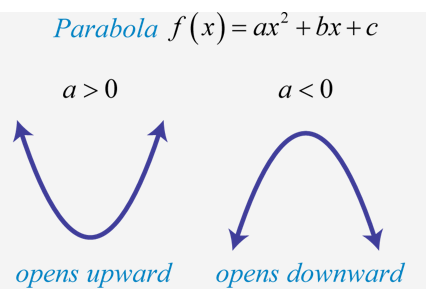## Summary

Generally form of a quadratic function $$f(x) = ax^2+bx+c$$

Standard form of a quadratic function $$f(x) = a(x - h)^2 + k$$

Hopefully, this blog has been successful in helping you understand how to graph a quadratic function and how to find the vertex of a quadratic function.

Points to Remember:-

• A polynomial function the degree of two which is known as a quadratic function.
• The graph of a quadratic function is also called a parabola. A parabola is a U-shaped curve it can open up or down.
• The axis of symmetry is the vertical line cross from the vertex.
• Quadratic functions are often written in a general form.
• The vertex can be found from an equation showing a quadratic function.
• The domain of a quadratic function is known as the all real numbers. The range varies with the function.

## About Cuemath

Cuemath, student-friendly mathematics and coding platform, conducts regular Online Live Classes for academics and skill-development, and their Mental Math App, on both iOS and Android, is a one-stop solution for kids to develop multiple skills.

Check out the fee structure for all grades and book a trial class today!

## What is the formula for the quadratic equation?

\begin{align} Ax^2 + Bx + C\end{align}

## Why is an equation having only two roots, one of which is raised to the second power, called a quadratic equation?

QUAD means four. when the equation is raised to 2, called a "QUADRATIC EQUATION"

## How to graph a quadratic function?

Step 1  Determine the y-intercept. To do this, set x=0 and find f(0).

Step 2 Determine the x-intercepts if any. f (x) = 0 and solve for x.

Step 3 Determine the vertex.

Step 4 We have at least five points to plot on either side of the line of symmetry.

Step 5 Plot the points and draw the graph. The points that we have found are:

How to find the vertex of a quadratic function?

$$y=x^2$$ Basic Squaring function

$$Y = (x - 2)^2$$ Horizontal shift right 2 units

$$Y = (x - 2)^2+3$$ Vertical shift up 3 units.

Use these translations to sketch the graph

## Which point is an x-intercept of the quadratic function $$f(x) = (x + 6)(x – 3)$$ ?

x=-6 and x=3 are the x intercept values.

(-6,0) and (3,0) are the x intercepts of the quadratic function $$f(x) = (x + 6)(x – 3).$$

## What are the zeros of the quadratic function $$f(x) = 8x2 – 16x – 15$$?

$$x = 1 + \sqrt\frac{23}{8}$$ or $$x = 1 - \sqrt\frac{23}{8}$$

## External References

-Written by Neha Tyagi, Cuemath Teacher

GIVE YOUR CHILD THE CUEMATH EDGE
Access Personalised Math learning through interactive worksheets, gamified concepts and grade-wise courses
Learn More About Cuemath